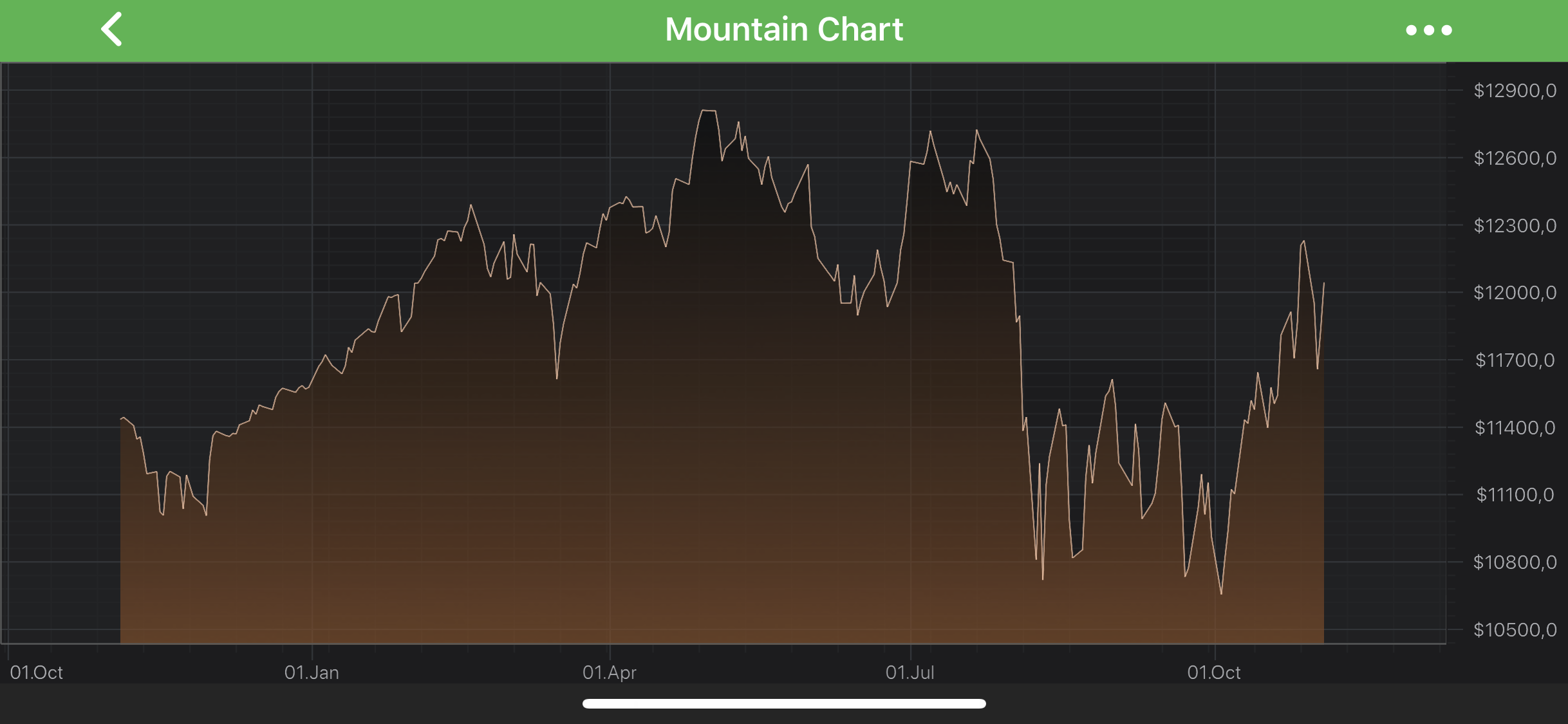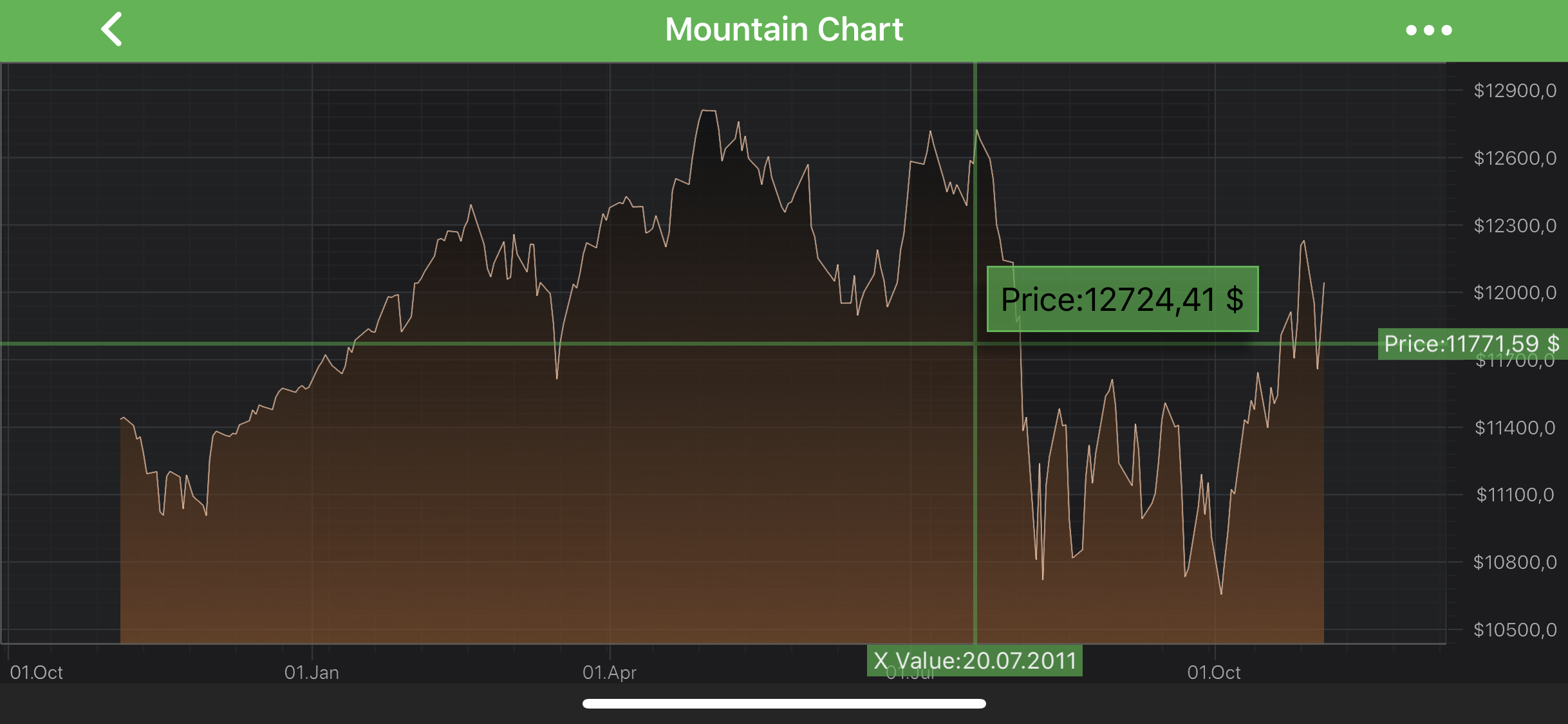iOS & macOS Charting Documentation - SciChart iOS & macOS Charts SDK v4.x

# Axis Labels - TextFormatting and CursorTextFormatting

All the axis classes obey standard Cocoa formatting strings, calling methods of the NSFormatter APIs internally. Thus, standard Date Formatters and Number Formatters strings patterns can be applied to format axis labels. There are the `ISCIAxisCore.textFormatting` and `ISCIAxisCore.cursorTextFormatting` properties for this purpose.

NOTE: `NSDateFormatter` and `NSNumberFormatter` relies on Unicode Technical Standard #35

See possible string patterns provided by UTS#35

## Axis Labels Formatting

`Axis API` allows to assign a formatting string for axis labels. There is the `ISCIAxisCore.textFormatting` property for this. Such formatting can be set in code as shown below:

id<ISCIAxis> xAxis = [SCIDateAxis new]; xAxis.textFormatting = @“dd.MMM”; id<ISCIAxis> yAxis = [SCINumericAxis new]; yAxis.textFormatting = @“\$0.0”;
let xAxis = SCIDateAxis() xAxis.textFormatting = “dd.MMM” let yAxis = SCINumericAxis() yAxis.textFormatting = “\$0.0”
var xAxis = new SCIDateAxis(); xAxis.TextFormatting = “dd.MMM”; var yAxis = new SCINumericAxis(); yAxis.TextFormatting = “\$0.0”;NOTE: Axis labels formatting is also applied to `SCIAxisMarkerAnnotation` labels.

## Axis Cursor Text Formatting

`Axis API` allows to assign a formatting string for axis overlays, such as `SCICursorModifier` axis labels. Similarly to axis labels formatting, there is the `ISCIAxisCore.cursorTextFormatting` property for this. Such formatting can be set in code as shown below:

id<ISCIAxis> xAxis = [SCIDateAxis new]; xAxis.cursorTextFormatting = @“‘X Value:'dd.MM.yyyy”; id<ISCIAxis> yAxis = [SCINumericAxis new]; yAxis.cursorTextFormatting = @“'Price:’###.##‘ \$’”;
let xAxis = SCIDateAxis() xAxis.cursorTextFormatting = “‘X Value:'dd.MM.yyyy” let yAxis = SCINumericAxis() yAxis.cursorTextFormatting = “'Price:’###.##‘ \$’”
var xAxis = new SCIDateAxis(); xAxis.CursorTextFormatting = “‘X Value:'dd.MM.yyyy”; var yAxis = new SCINumericAxis(); yAxis.CursorTextFormatting = “'Price:’###.##‘ \$’”;## Numeric Axes and Scientific Notation

It is possible to render axis labels and text in tooltips in a shortened form using scientific notation (standard form). This becomes an issue when working with large numbers. In this case axis size will grow to fit axis labels inside. This is a common issue when working with `SCILogarithmicNumericAxis`.

To configure an axis to show numbers in this form, it is necessary that a proper format string is provided and `SCIScientificNotation` is set to the desired value. There is a `ISCINumericAxis.scientificNotation` property for this purpose. Possible options are listed below:

• `SCIScientificNotation.SCIScientificNotation_None` - the default value.
• `SCIScientificNotation.SCIScientificNotation_Normalized` - assumes 10 as base.
• `SCIScientificNotation.SCIScientificNotation_E` - assumes the number E as base.
• `SCIScientificNotation.SCIScientificNotation_LogarithmicBase` - used with `SCILogarithmicNumericAxis`. Assumes the logarithmic base of the `LogarithmicNumericAxis` as base.

An axis can be configured to use `SCIScientificNotation` like follows:

id<ISCIAxis> xAxis = [SCILogarithmicNumericAxis new]; xAxis.logarithmicBase = 10.0; xAxis.scientificNotation = SCIScientificNotation_LogarithmicBase; xAxis.textFormatting = @“#.#E+0”; xAxis.cursorTextFormatting = @“#.#E+0”;
let xAxis = SCILogarithmicNumericAxis() xAxis.logarithmicBase = 10.0 xAxis.scientificNotation = .logarithmicBase; xAxis.textFormatting = “#.#E+0” xAxis.cursorTextFormatting = “#.#E+0”
var xAxis = new SCILogarithmicNumericAxis(); xAxis.LogarithmicBase = 10.0; xAxis.ScientificNotation = SCIScientificNotation.LogarithmicBase; xAxis.TextFormatting = “#.#E+0”; xAxis.CursorTextFormatting = “#.#E+0”;## Dynamically Changing TextFormatting

For more advanced formatting scenarios, `Axis API` provides a feature called LabelProviders. It grants full control over text output of every axis label. This can be useful if required to customize textual representation of particular axis labels or replace all of them with other strings based on some logics. Please refer to the LabelProvider API article for further details.